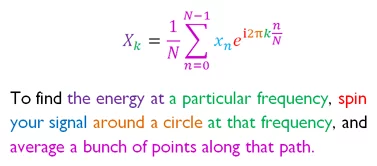by Seungjae Ryan Lee

To those unfamiliar with the topic, math equations can be confusing. If not explained properly, it is difficult to understand the meaning behind the equation without scratching one’s head a few times. Some nice way to make such equations more “digestible” is to color different components, like this explanation of Fourier Transform:Each component is color-coded, allowing the reader to easily understand which part of the explanation corresponds to which part of the equation. Unfortunately, for complex equations like this, the number of colors increase, and having 7 colors like this is a bit “wild”. Also, it does not help those that are colorblind.

MathCaptions is a JavaScript library that allows you to highlight parts of equation that match the explanation. Instead of trying to highlight all components at once, it highlights each component individually, allowing for a cleaner and colorblind-friendly interface.

Here is the same explanation of Fourier Transform with MathCaptions. Try hovering on the equation or the explanation!

$\class{mc-math mc-energy}{X}_\class{mc-math mc-frequency}{k} = \class{mc-math mc-bunch}{\frac{1}{N} \sum^{N-1}_{n=0}} \class{mc-math mc-signal}{x_n} \class{mc-math mc-spin}{e}^{\class{mc-math mc-spin}{i} \class{mc-math mc-circle}{2\pi} \class{mc-math mc-frequency}{k} \class{mc-math mc-bunch}{\frac{n}{N}}}$
To find the energy at a particular frequency, spin your signal around a circle at that frequency, and average a bunch of points along that path.

## How To Use MathCaptions

MathCaptions can be used with MathJax. Copy below JavaScript code to a new file and import it at the header of your HTML file.

Full Code for MathCaptions v1.0
1
2
3
4
5
6
7
8
9
10
11
12
13
14
15
16
17
18
19
20
21
22
23
24
25
26
27
28
29
30
31
32
33
34
35
36
37
38
39
40
41
42
43
44
// Initialize MC with jQuery logic
function setupMC() {
for (let mcMath of $(".mc-math")) { // Find class of type mc-[name] let classList =$(mcMath).attr("class").split(/\s+/);
let mcCaption = null;
for (let className of classList) {
let matches = /^mc\-(.+)/.exec(className);
if (matches != null && matches != "mc-math") {
// Redefine mcMath since if there are multiple with same name, we should highlight them all
mcMath = $(.mc-math.${matches});
mcCaption = $(.mc-caption.${matches});
break;
}
}

// Insert hover logic
if (mcCaption != null) {
$(mcMath).hover( function() {$(mcMath).css({"font-weight": "bold"});
$(mcCaption).css({"font-weight": "bold", "text-decoration-line": "underline"}); }, function() {$(mcMath).css({"font-weight": "normal"})
$(mcCaption).css({"font-weight": "normal", "text-decoration-line": "initial"}) } );$(mcCaption).hover(
function() {
$(mcMath).css({"font-weight": "bold"});$(mcCaption).css({"font-weight": "bold", "text-decoration-line": "underline"});
},
function() {
$(mcMath).css({"font-weight": "normal"})$(mcCaption).css({"font-weight": "normal", "text-decoration-line": "initial"})
}
);
}
}
}
MathJax.Hub.Queue(function() {
setupMC();
});


You can are now ready to use MathCaptions!

To highlight components, you need to choose a name for each component and label the correct parts of the equation and the description. Then, add classes .mc-math.mc-[name] to the component in the equation, and add .mc-caption.mc-[name] to the same component in the description. MathCaptions will take care of the rest!

To illustrate, here is a demo of a simple description of the AM-GM inequality.

1
2
3
4
5
6
7
$$\class{mc-math mc-am}{\frac{x+y}{2}} \class{mc-math mc-geq}{\geq} \class{mc-math mc-gm}{\sqrt{xy}}}$$

<span class="mc-caption mc-am">The arithmetic mean</span> of two non-negative
real numbers <span class="mc-caption mc-geq">is greater than or equal to</span>
the <span class="mc-caption mc-gm">geometric mean</span> of them.


This HTML block will be rendered like this:

$\class{mc-math mc-am}{\frac{x+y}{2}} \class{mc-math mc-geq}{\geq} \class{mc-math mc-gm}{\sqrt{xy}}$
The arithmetic mean of two non-negative real numbers is greater than or equal to the geometric mean of them.